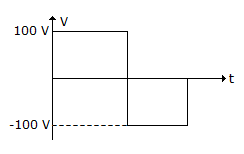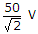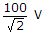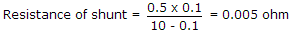# Electronics and Communication Engineering - Measurements and Instrumentation

### Exercise :: Measurements and Instrumentation - Section 1

31.

A full wave rectifier type meter is used to measure the wave shape shown in figure. The voltmeter is calibrated to read rms value of sine wave. The reading of voltmeter will beA.B.C. 100 V D. 111 V

Explanation:

Peak value of sine wave = 100 x 1.11 = 111 V.

32.

A moving coil instrument has a resistance of 0.5 Ω and a full scale deflection of 0.1 A. To convert it into an ammeter of 0-10 A the shunt resistance should be

 A. 0.004 Ω B. 0.005 Ω C. 0.05 Ω D. 0.1 Ω

Explanation:.

33.

Potentiometer method of dc voltage measurement is more accurate than direct measurement using a voltmeter because

 A. it loads the circuit to maximum extent B. it loads the circuit moderately C. it does not load the circuit at all D. it uses centre zero galvanometer instead of Voltmeters

Explanation:

Since the circuit is not loaded, the accurate value of dc voltage is measured.

34.

Assertion (A): A hot wire ammeter has a cramped scale.

Reason (R): The heat is proportional to square of current.

 A. Both A and R are true and R is correct explanation of A B. Both A and R are true but R is not correct explanation of A C. A is true R is false D. A is false R is true

Explanation:

Since heating ∝ I2, scale is cramped.

35.

Which of the following is true about an impulse voltage?

 A. It is a unidirectional voltage B. Chopped impulse flashover does not occur C. Time taken to rise is equal to time taken to fall D. RMS value is always less than 50% of average value